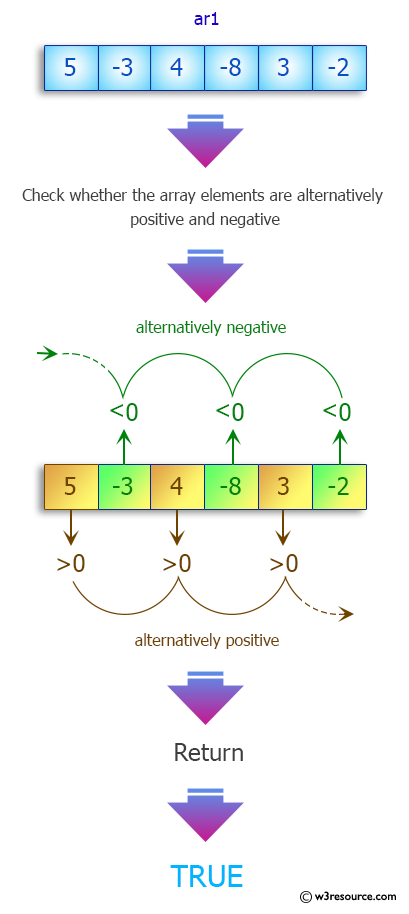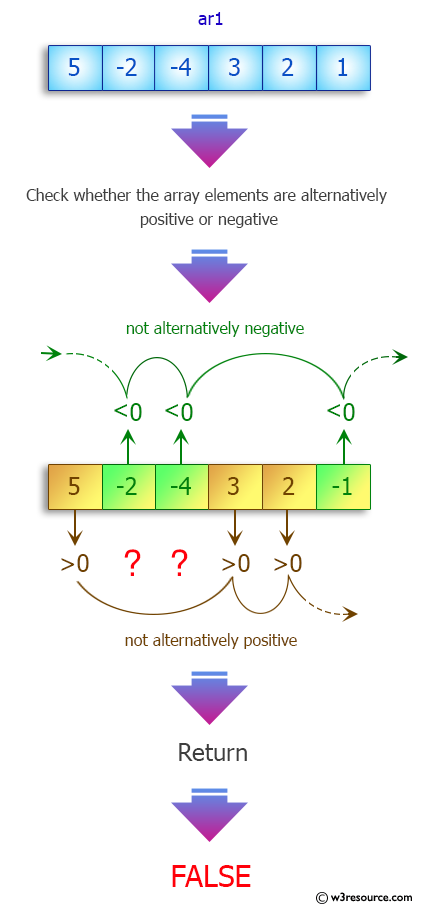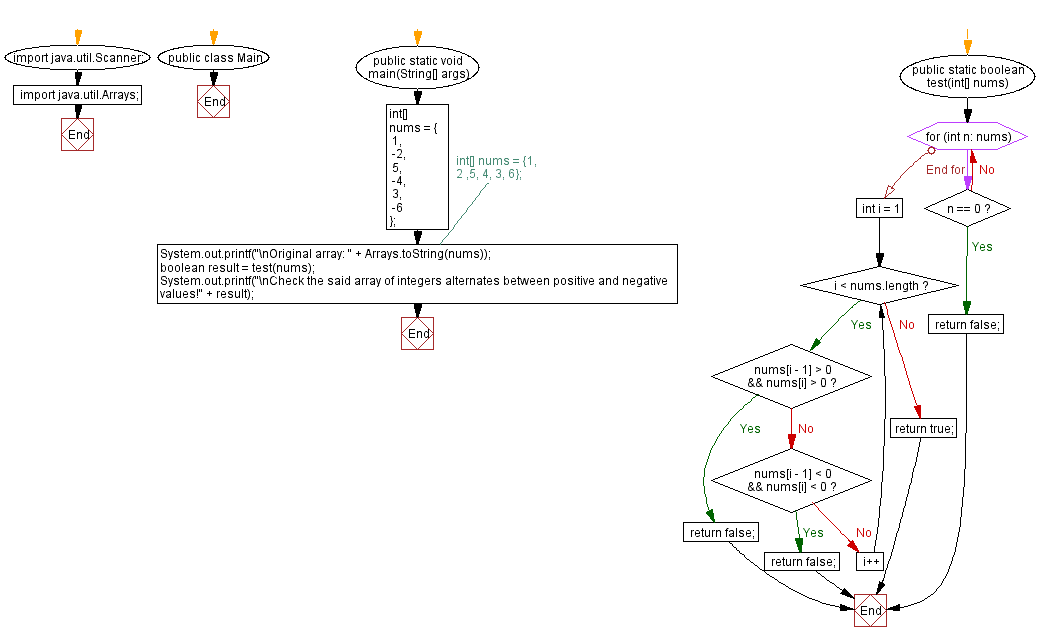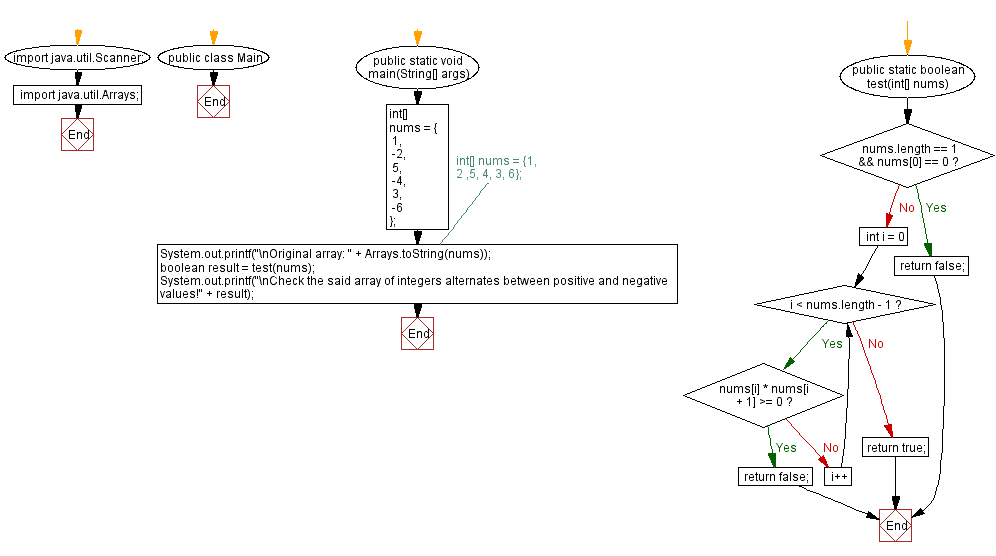﻿ Java - Positive and negative values alternate in an array

# Java: Positive and negative values alternate in an array

## Java Array: Exercise-77 with Solution

Write a Java program that checks whether an array of integers alternates between positive and negative values.

Sample Data:
{1, -2, 5, -4, 3, -6} -> true
{1, 2 ,5, 4, 3, 6} -> false

Pictorial Presentation:Sample Solution-1:

Java Code:

``````// Import necessary Java classes.
import java.util.Scanner;
import java.util.Arrays;

// Define the 'Main' class.
public class Main {
// Define the main method for running the program.
public static void main(String[] args) {
// Initialize an array of numbers.
int[] nums = {1, -2, 5, -4, 3, -6 };
// Alternatively: int[] nums = {1, 2 ,5, 4, 3, 6};
System.out.printf("\nOriginal array: " + Arrays.toString(nums));

// Call the 'test' method to check if the array alternates between positive and negative values.
boolean result = test(nums);
System.out.printf("\nCheck the said array of integers alternates between positive and negative values! " + result);
}

// Define the 'test' method to check if the array alternates between positive and negative values.
public static boolean test(int[] nums) {
// Iterate through the elements in the 'nums' array.
for (int n: nums) {
// If any element is zero, return false.
if (n == 0)
return false;
}
// Iterate through the 'nums' array, starting from the second element.
for (int i = 1; i < nums.length; i++) {
// Check if there are consecutive positive or negative numbers; return false if found.
if (nums[i - 1] > 0 && nums[i] > 0) {
return false;
} else if (nums[i - 1] < 0 && nums[i] < 0) {
return false;
}
}
// If no consecutive positive or negative numbers are found, return true.
return true;
}
}
```
```

Sample Output:

```Original array: [1, -2, 5, -4, 3, -6]
Check the said array of integers alternates between positive and negative values!true
```

Flowchart:Sample Solution-2:

Java Code:

``````// Import necessary Java classes.
import java.util.Scanner;
import java.util.Arrays;

// Define the 'Main' class.
public class Main {
// Define the main method for running the program.
public static void main(String[] args) {
// Initialize an array of numbers.
int[] nums = {
1,
-2,
5,
-4,
3,
-6
};
// Alternatively: int[] nums = {1, 2 ,5, 4, 3, 6};
System.out.printf("\nOriginal array: " + Arrays.toString(nums));

// Call the 'test' method to check if the array alternates between positive and negative values.
boolean result = test(nums);
System.out.printf("\nCheck the said array of integers alternates between positive and negative values! " + result);
}

// Define the 'test' method to check if the array alternates between positive and negative values.
public static boolean test(int[] nums) {
// Check if the array contains a single element with the value 0; return false in this case.
if (nums.length == 1 && nums == 0)
return false;
// Iterate through the 'nums' array.
for (int i = 0; i < nums.length - 1; i++)
// Check if the product of adjacent elements is non-negative (i.e., both positive or both negative); return false in this case.
if (nums[i] * nums[i + 1] >= 0)
return false;
// If no consecutive elements with the same sign are found, return true.
return true;
}
}
```
```

Sample Output:

```Original array: [1, -2, 5, -4, 3, -6]
Check the said array of integers alternates between positive and negative values!true
```

Flowchart:Java Code Editor:

Previous Java Exercise: Consecutive Numbers in an array.
Next Java Exercise: Negative Dominant.

What is the difficulty level of this exercise?

Test your Programming skills with w3resource's quiz.

﻿